Currently there is no translation for this activity.

# Where on Earth am I?

Created: 2018-02-26
Author(s): Markus Nielbock, Haus der AstronomieGoals

The students will understand the basic principle behind satellite-based positioning and navigation. Using a simulated and simplified 2-D model of the satellite configuration, they will learn to determine their location on Earth solely by translating the signal travel time received from four satellites. As an additional task, the students will correct the a priori unknown temporal offset of the receiver clock. As a result, the students will also learn or recall the locations of European countries.Learning Objectives

At the end of the lesson, students will be able to: - locate and name the European countries - name the two main global navigation satellite systems - describe the basic functionality of a global navigation satellite system - calculate the length of a signal travel path based on the signal travel time and the speed of light - calculate the mean of four numbers - explain why the face values of signal travel times from the GPS satellites lead to a deviation from the true positionBackground

Navigation is an old technique that allows a person to locate a position on Earth. For this, one needs known reference points from which one’s own position can be derived. Satellite navigation provides moving references, i.e. satellites. The most prominent satellite navigation system is the Global Positioning System (GPS) (see corresponding section below). While few know how it actually works, most people know that is used in car navigation and in modern smartphones.

To derive one’s own location on Earth, the basic step is determining the distance to the satellites. From this, the desired location can be calculated. This technique is called trilateration. To measure the distance to those satellites, the constant speed of light is used. If one knows the duration a signal takes to travel from the source to the receiver, the known velocity directly yields the distance.

An analogue to this technique is estimating the distance to a thunderstorm by noting the time between a flash of lightning and the sound of thunder. Since lightning arrives almost instantly, the travel time of the following thunder can be converted into distance. The conversion is done by using the sound speed. Under normal circumstances, the sound of thunder travels 1 km in approximately 3 seconds (v = 343.2 m/s). If several observers calculate the interval between lightning and thunder from different positions, the location of the thunderstorm can be determined.

The challenge with satellite navigation is to exactly measure the signal travel time. Each transmitted signal contains a code that provides information about the corresponding satellite, its position and the time of transmission. Each satellite is equipped with an extremely exact and synchronised atomic clock. On receiving the signal, the clock inside the GPS receiver helps calculate the time the signal needed to travel.

Imagine a satellite sends a signal. After a given duration, the signal will have covered the same distance in all directions. Hence, it is transmitted on the surface of a sphere.

Figure 1: Configuration of two satellites. Position A on Earth receives the signals after 4 and 5 seconds, respectively. The two signals intersect at points A and B. Since only A is on Earth, B can be discarded (own work).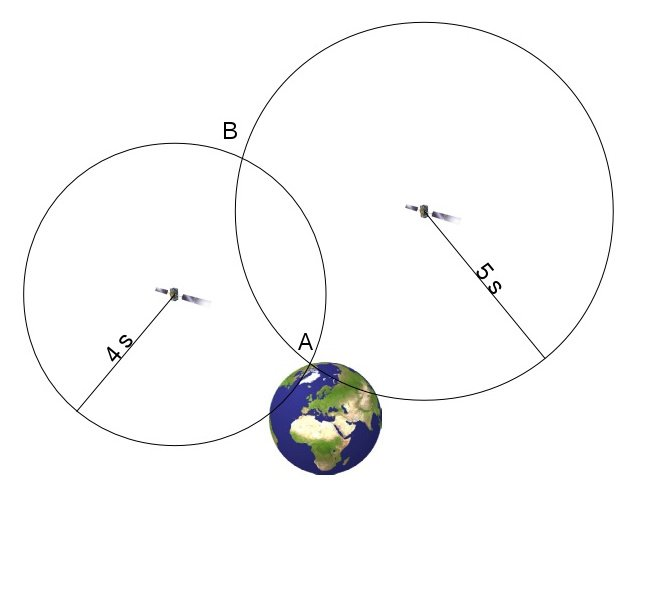For the rest of this activity, the three-dimensional configuration of Earth and the satellites will be represented by simplified two-dimensional illustrations. The surface of the satellite signal wave front will be depicted by circles instead of spheres. Consequently, the intersections of two satellite signals are points instead of lines or arcs. This is done to improve the visibility of the illustrations.

The principle of positioning is shown in Figure 1. At an unknown position on Earth, the signals of the two satellites arrive at 4 and 5 seconds, respectively. Therefore, the location must be at one of the two intersections. Since only A is on Earth, position B can be discarded. However, this only works well, if the signal travel time is measured very accurately. Unfortunately, the clocks of the GPS receivers are usually quite inaccurate. If, for example, the receiving clock is 0.5 s fast, the measured intersection is A’ instead of A in Figure 2. The positioning is equally inaccurate. Therefore, at this step, the term pseudorange is introduced, which is the assumed distance to one of the satellites derived from the face values of time measurement.

Figure 2: The same configuration as in Figure 1, but this time with a receiving clock running 0.5 s fast. Therefore, the signal transmission times are measured to be 0.5 s longer. This results in an intersection at A' instead of A (own work).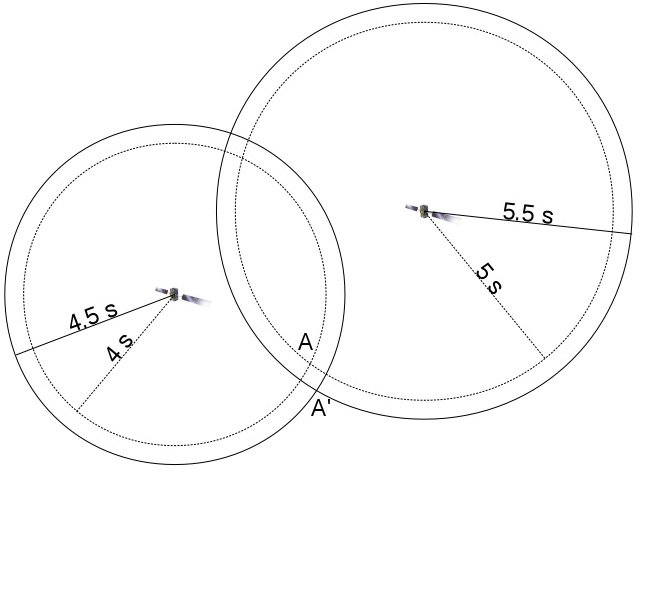This error can be reduced by adding another satellite (Figure 3). Again, the signal travel time is erroneously measured as 0.5 s later. This configuration is different from the previous situation with two satellites as it produces three intersections. It now becomes obvious that the measurement is inaccurate, and the true position must be surrounded by the apparent ones.

The GPS receiver notices the incompatible pseudoranges. It initiates an algorithm that changes the time of the receiver clock until a common intersection is found, or the difference between the pseudoranges is minimised. The GPS receiver clock can be corrected accordingly, and that speeds up the following positioning attempts. Each additional satellite improves the precision.

As mentioned before, this illustration uses a 2-D configuration. In reality, at least four satellites are needed to locate a position on Earth with acceptable accuracy.

Figure 3: A third satellite is added, whose signal travel time is again measured wrongly. This leads to three intersections A' surrounding the true position A (own work).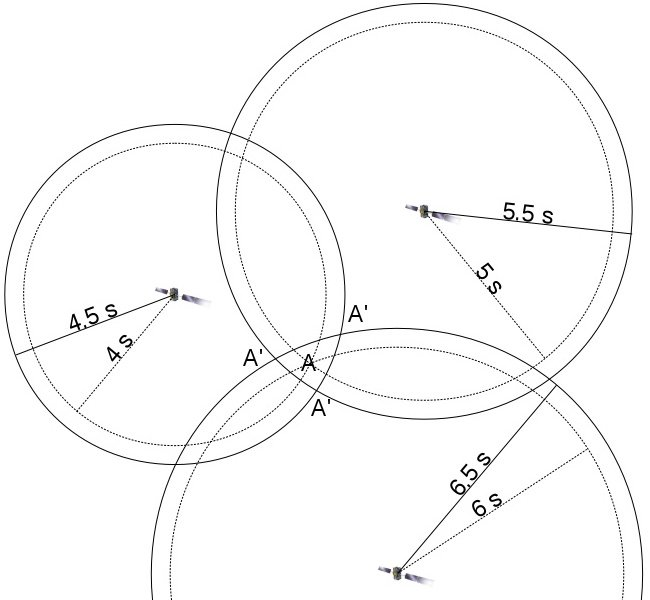Modern applications of satellite navigation
Initially, satellite navigation was developed for military purposes. Today, it is crucial for many modern commercial businesses and transport services. Often, we do not even notice that satellite positioning is involved. Different navigational techniques that were introduced for a large variety of transport vessels have now been replaced by satellite navigation (cars, trucks, ships, airplanes). They play a role in services as simple as package tracking and as complex as helping businesses distribute their goods. Satellite navigation can also help save lives; for example, an autonomous emergency system can automatically transmit the location of a car that has been involved in an accident.

GPS and Galileo
Global systems of satellites used for positioning and navigation are called global navigation satellite systems (GNSS). The most renowned GNSS is the GPS (Global Positioning System), or officially Navstar GPS. It was developed by the US military in the 1970s. Currently, it consists of 32 satellites, of which at least 24 are always operational. They are orbiting Earth at an altitude of 20200 km and on 6 trajectories inclined with respect to each other.

Although it was originally designed for military purposes, its full capabilities have been available for general and civil use since 2000. Nevertheless, the USA holds the right to artificially reduce the accuracy of the GPS for tactical reasons at any time. Since many civilian applications rely on fully working services of the GPS, they are potentially in constant danger to fail.

This is one reason, and not necessarily the least, why the European Union (EU) decided to develop their own GNSS called Galileo, to be controlled by civilian authorities. However, it is also intended to serve tactical, security and defence purposes. Galileo will be compatible with other GNSS like the US-based GPS, the Russian GLONASS as well as the Chinese Beidou.

At the same time, the USA is currently working on a third generation of the GPS. The new satellites will be equipped with an additional signal band that makes them compatible with the Galileo system. Moreover, they will lack the option to intentionally reduce the accuracy of the positioning.

In 2017, the fleet of 30 Galileo satellites, of which 6 are spares, is still incomplete. They will be in three orbits inclined with respect to one another and at an altitude of 23222 km. First services were initiated in 2016. Full operations are expected to start in 2020.

Figure 4: Illustration of the satellite orbits of the Galileo GNSS (Credit: ESA/P. Carril).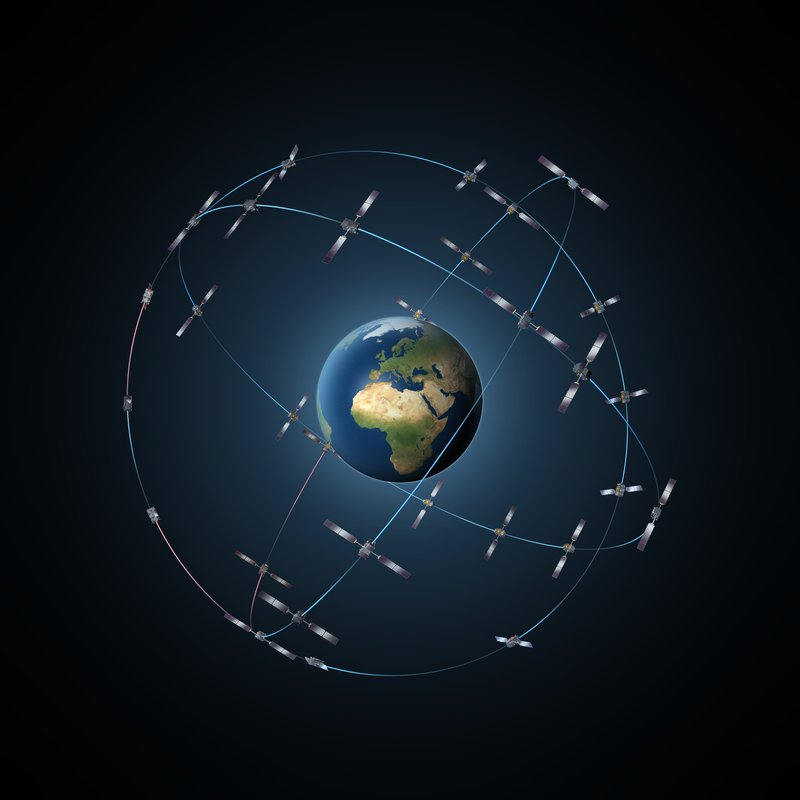The accuracy of Galileo is expected to be of the order of 4 m without any additional corrections. This is about 3 times better than what the GPS can achieve. Galileo can reach higher accuracies and precisions by including terrestrial reference stations (differential GPS). In this way, Galileo will be accurate enough for applications in aerial, marine and land navigation. In common car navigation devices, it will be possible to determine the driving lane.

Figure 5: Computer image of a Galileo satellite (Credit: ESA/P. Carril).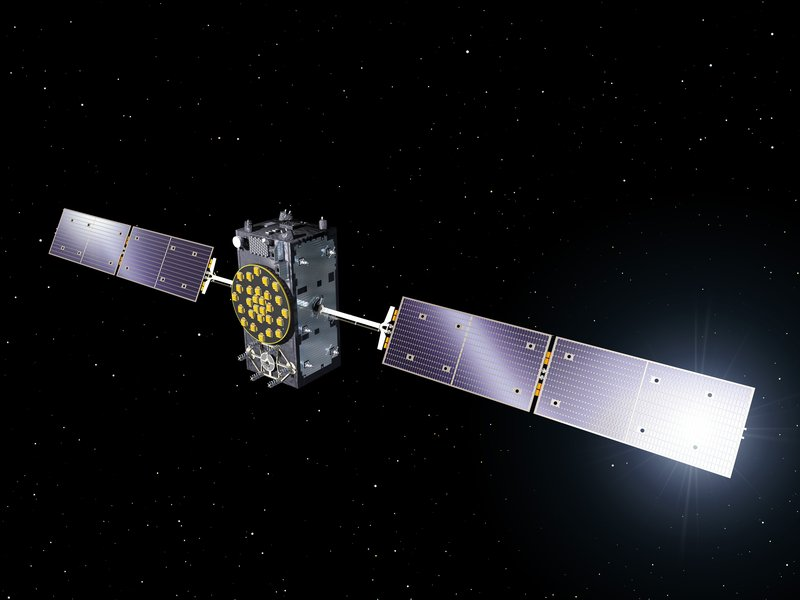Signal speed
Radio signals are electromagnetic waves that travel at the speed of light. In vacuum conditions, its value is 299792458 m/s, according to the BIPM (Bureau International des Poids et Mesures).

Graphical approximation of centre of mass
At the end of this activity, the students have to determine their location inside an area of intersection constructed by arcs drawn with compasses. A real GPS receiver runs an interpolation algorithm to determine the location and the clock correction. The students will use a simpler approach that approximates the correct result.

Given is an irregularly shaped area confined by four lines (Figure 6). The first step is to determine the bisectors of each of the four lines. In the second step, the opposing bisectors are connected with lines. This results in two crossing lines whose intersection is an approximation of the centre of mass.

Figure 6: Illustration of how to approximate the centre of mass of an irregular tetragon. The shape is confined by four lines (left). After finding the bisectors of each line, the opposing bisectors are connected with a line (right). The intersection is an approximation of the centre of mass (own work).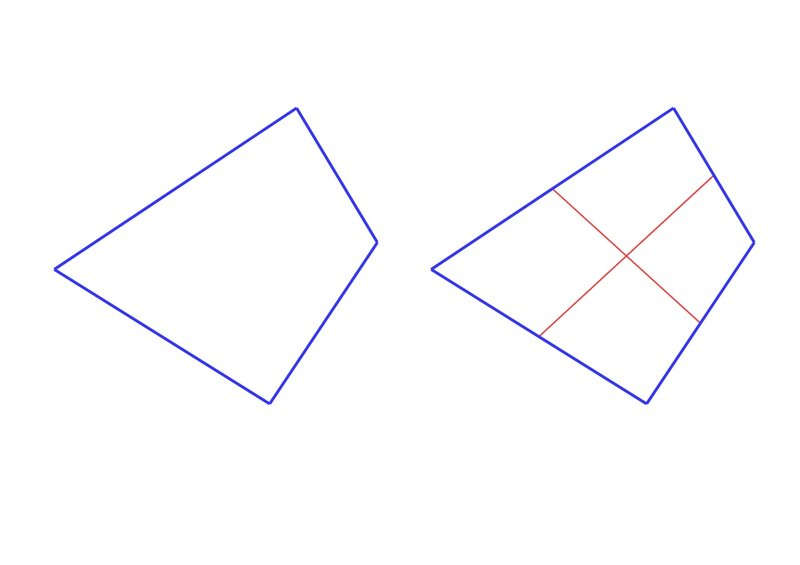Average
An average value is defined as a number that is the minimum of the sum of differences between the average and the individual values. In statistics, it is used to calculate a representative number when many values that scatter around the true value.

If t stands for a given value of a time measurement, we can indicate a series of measurements by adding an index, like e.g. t1, t2, t3, … which corresponds to the first, second and third value, respectively. In order to calculate the average of a series of measurements, one has to calculate the sum of the individual values and divide it by the number of measurements. For three temperature measurements, the average would be calculated as follows: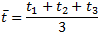Or in general: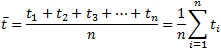t ̅ is the symbol for the average of times measured, and n is a natural number that corresponds to the number of measurements.

Glossary
Trilateration
Navigational technique to determine a position by measuring the distances to at least three reference points.

Pseudorange
The calculated distance to the reference points used in trilateration without applying the necessary corrections. The pseudorange is purely based on the face values of the underlying measurement.

Bisector
The mid-point of a straight line.Materials

The list contains the items needed by each student. Some of them can be shared between two to four students. - Worksheets - Compasses (drawing tool) - Pencil - Ruler (at least 20 cm) - CalculatorFull Description

### INTRODUCTION

Introduce the topic by asking the students if they had heard of the GPS (they most probably do). Ask them where they use GPS. Almost every modern mobile phone has a GPS receiver.

Tell them about the different GNSSs. At the end, let them summarise the content.

Ask the students if they can explain how measuring the time of a signal transmitted by the satellites provides the distance to it? Help them by bringing up the analogue with the thunderstorm. There is typically at least one student that knows about it. See the introduction for details.

Ask them how counting too fast/slow changes the result.

Tell them that with the satellites, they will have to measure the travel time of radio signals, a special kind of light, instead of the sound of the thunder. Ask them if they had an idea of how one could determine the signal travel time of a given satellite. The answer contains clocks to measure time.

Optional:
After having established that those signals are transmitted by satellites, you may want to show them where and how many satellites there are. This can be done via free apps for smartphones like e.g. GPS Essentials. However, receiving GPS signals within buildings may not be easy.

Android:

Apple iPhone:
https://itunes.apple.com/de/app/ultimate-gps/id403066634?mt=8

### ACTIVITY 1 (OPTIONAL): IDENTIFYING EUROPEAN COUNTRIES

Materials: - Worksheet 1 - Pencil

During the actual exercise, where the students locate their simulated position, they have to use a map of Europe. As an additional task, the teacher can ask them to identify European countries on a blank version of that map.

Hand out worksheet 1, which is the same as the map used in the next activity, but without the names of the countries. If available and necessary, hand out atlases.

As an additional optional exercise, let the students name the capitals of those countries.

In a culturally diverse classroom, the students may want to talk about the countries where they or their ancestors come from.

Figure 7: Topographical map of Europe displayed in Lambert azimuthal equal-area projection. This assures a constant scale throughout the entire map. The country borders are indicated with grey lines (Alexrk2, https://commons.wikimedia.org/wiki/File:Europe_relief_laea_location_map.jpg, ‘Europe relief laea location map’, https://creativecommons.org/licenses/by-sa/3.0/legalcode).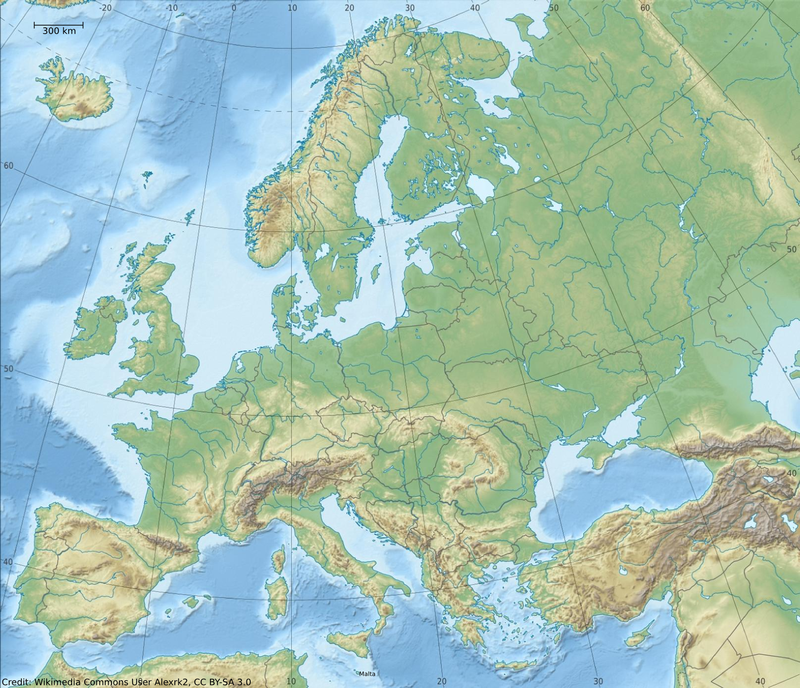The results can be checked with the map handed out in the next activity.

### ACTIVITY 2: LOST IN NO-MAN'S LAND

Materials: - Worksheet 2 - Compasses (drawing tool) - Pencil - Ruler (at least 20 cm) - Calculator

Hand out Worksheet 2 and repeat the story given below:

Imagine, you were abducted by aliens and taken on a ride across the solar system. On your return, you were dropped off somewhere in Europe. You have no idea where you are, but luckily you have your GPS receiver with you that should guide you to a place from where you can receive help or return home.
But … oh no … the receiver is broken. Instead of showing your location on Earth, it only displays the signal travel time of four receiving satellite signals. You will have to do it all by yourself.
Since the clock in the receiver is not perfect, the time on the display can be off from the true value. But you can deal with that later. With the map you find in your pocket and the working calculator function of the GPS receiver, you should be able to get along.

Figure 8: Topographical map of Europe displayed in Lambert azimuthal equal-area projection. This assures a constant scale throughout the entire map. On Worksheet 2, the scale is represented as follows: 300 km = 1.3 cm on the map. The names of the countries have been added (for credits see Figure 7).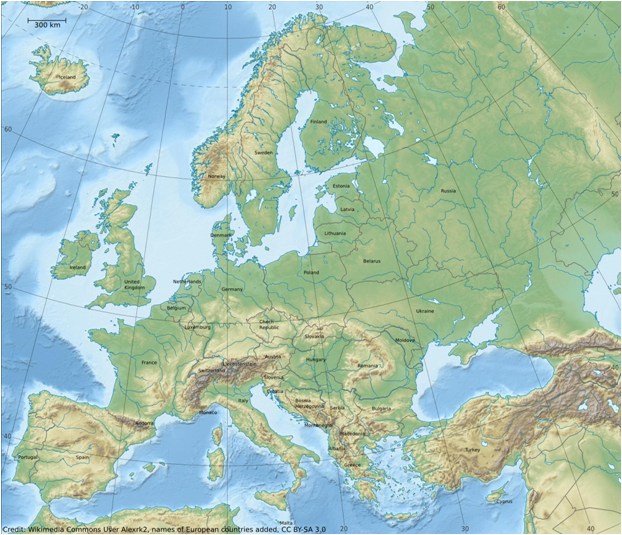(Note: The map provided is only a 2-D model of the real configuration, i.e. the altitude of the satellites above the ground is neglected.)

In the first step, the students use information on their worksheets to determine their approximate location. The signal travel times in the worksheet table (see Table 1) deviate contain an offset from the correct time, simulating a GPS receiver clock that is several milliseconds fast.

Instructions
The time the signals take to reach to you is given in milliseconds (1000 ms = 1 s).

Radio signals are transmitted at the speed of light, so you only have to use the constant value of that speed (c = 299792.458 km/s) to convert the time into a distance.

Example:
Let us assume that the measured signal travel time is 10 ms (milliseconds). The measured distance to the satellite can be calculated as follows:

Δs = c • Δt

Δs: distance to the satellite
c: speed of light
Δt: signal travel time

Δs = 299792.458 km/s • 10 ms = 299792.458 km/s • 0.01 s = 2997.92 km

Instruct the students to always translate the time from milliseconds into seconds.

What is the scale of the map?
The first step is to determine the scale of the map. This should be done as accurately as possible. There is a line indicating 300 km. Important: Do not change the scale of the map in the worksheets. It indicates that 300 km on land correspond to 13 mm on the map.

How far away are the satellites?
Explain that the students should use the table on the worksheet (Table 1) by filling in the missing numbers. For this part of the activity, only the columns labelled ‘measured’ will be used. The columns labelled ‘corrected’ are only needed for the additional activity for advanced students (see below).

Table 1: Table as provided in Worksheet 2.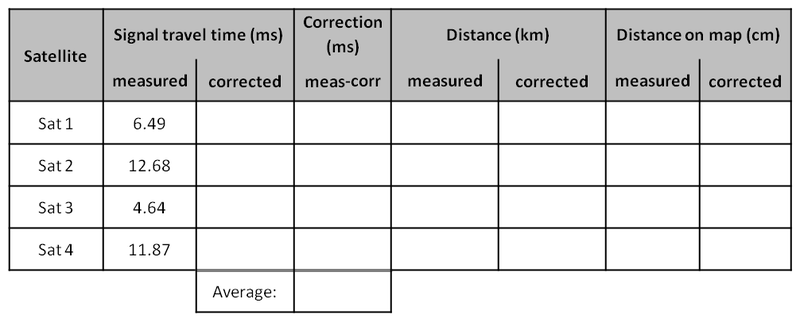Now, the students are supposed to determine the distances the signals have travelled from the time displayed on the receiver (see table). Minding the units, the times have to be multiplied by the constant speed of light (299792.458 km/s). The calculated values are entered under the column ‘Distance (km), measured’.

Using the scale of the map, the students convert this distance to the one on the map. As mentioned before, 300 km on Earth correspond to 13 mm on the map. This value is then added under the column ‘Distance on map (cm), measured’. The resulting values are given in Table 2.

The students then use their compasses and draw circles or arcs with radii equal to the signal travel lengths. With each additional arc, the students are supposed to determine the countries they are in.

Question: What do you notice about your likely position with each additional satellite signal arc?
Answer: With each additional satellite arc, the position is determined with a higher precision.

After drawing the arc for the last satellite, the students will see that the four arcs do not intersect in a single point, but in an area that should include the true location (Figure 9). This is due to the problems in the receiver clock. The correct position is somewhere in the area enclosed by the intersections.

Question: What are the possible countries of your current position?
Answer: Possible solutions are the Netherlands, Belgium, Luxemburg and Germany.

Figure 9: Topographical map of Europe displayed in Lambert azimuthal equal-area projection. The arcs represent the distances that the signals have travelled from the four satellites in the times specified in the table of Worksheet 2 (for credits see Figure 7).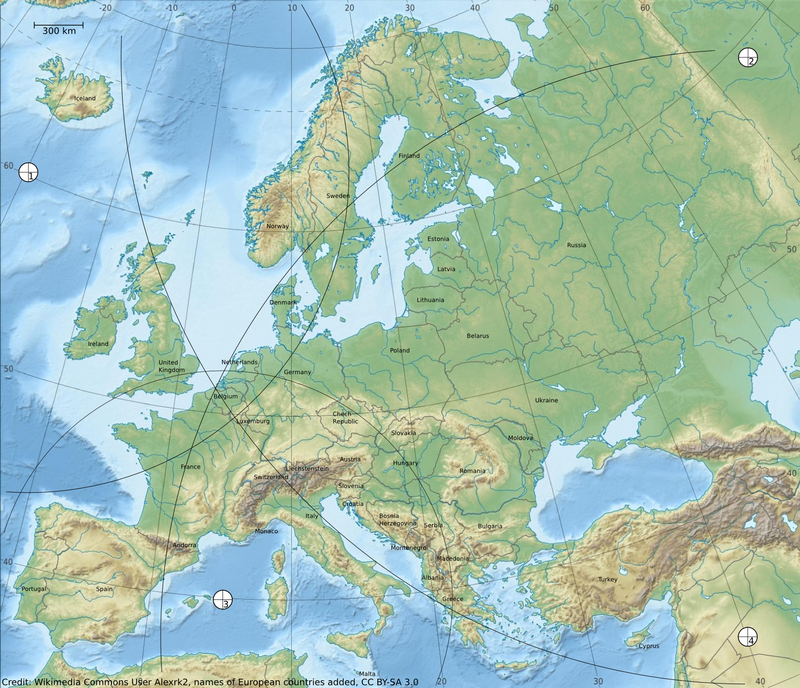To determine the location more precisely, e.g. in order to be prepared for the extension activity below, a single point must be identified that represents the true location. Two approximations can be used for this.

Simple solution
The centre of mass of the resulting area of intersection can be estimated by guessing a point that has the same distance from all four intersections of pairs of arcs. It should be in the southern part of the Netherlands.

In real life, GPS receivers change the time offset common to all receiving signals until a point of intersection is found or the location is narrowed down to an small area. This algorithm can be simplified in this example by approximating the centre of mass graphically. The common area is surrounded by four arcs. The students have to determine the bisectors for each of them. Then, they connect the opposing bisectors with lines. This results in two crossing lines whose intersection can be defined as the centre of mass (Figure 6). It is a good approximation of the true location. Again, the location should be in the southern part of the Netherlands (Figure 10).

Figure 10: The same map as before, but this time with the approximation for the simulated location (for credits see Figure 7).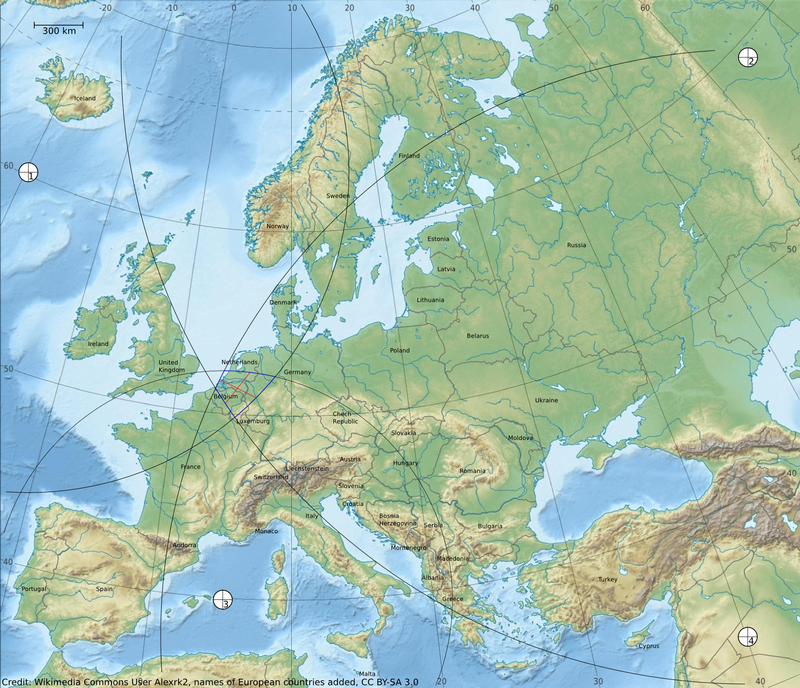### EXTENSION FOR ADVANCED STUDENTS: CORRECTING THE CLOCK

Question: What is the reason for the mismatch between the distances derived from the GPS measurements and the location determined by interpolation?
Answer: The clock of the GPS receiver is incorrect.

In a real GPS receiver, this interpolated position is used to calculate a correction for the satellites. This is then assumed for subsequent positioning. This additional activity illustrates these calculations and determines the offset of the receiver clock.

After determining the centre of mass of the area of intersection (Figure 10), the students measure its distance to each of the satellites on the map. The values are added to the table on their worksheets in the column labelled ‘Distance on map (cm), corrected’. These numbers are then converted into true distances using the map scale and added to the table in column ‘Distance (km), corrected’.

The corrected signal travel times for each of the satellites are calculated using the speed of light and added to column ‘Signal travel time (ms), corrected’. With this, the students calculate the difference between the initial value given by the simulated GPS receiver and the corrected one. These differences are added to the column ‘Correction (ms), meas-corr’. From the resulting four differences, the students derive the average. This is the clock correction the simulated GPS receiver would have to apply to any additional measurements. The resulting numbers would be similar to the ones in Table 2.

Table 2: Table with results.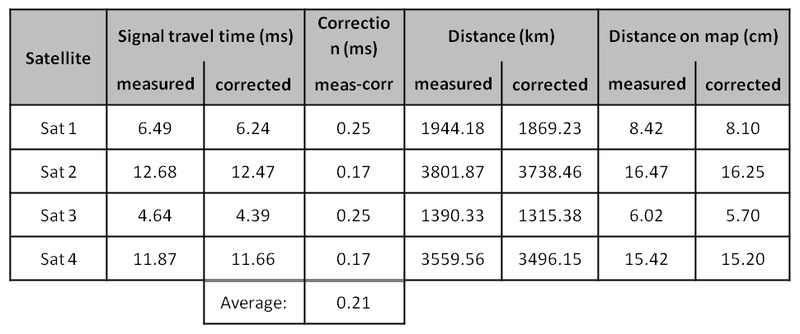Note that due to uncertainties introduced by the drawing on the map, the numbers can vary a bit.Evaluation
• Ask the students to identify the European countries shown on the map in Activity 1.
• When introducing the topic, talk about GPS and Galileo. Afterwards, ask the students to summarise the concept of global navigation satellite systems.
• Ask the students how they can infer the distance to a thunderstorm (counting the seconds between the lightning and the thunder and dividing that number by three). Ask them how that would work if the information about a satellite’s distance in space was transmitted via light (or radio signals) instead of sound.
• In Activity 2, ask the students to demonstrate this ability by solving the task.
• Ask the students why in the end, the intersection of all the satellites is an area and not a single point. Which of the initial data could be wrong and why?Curriculum

Germany

Country/ Fed. State | School | Level | Subject | Section --- | --- | --- | --- D/BW | Sek I, Gym, OS Gem | All | NWT | 2.1 Erkenntnisgewinnung und Forschen
2. Bestimmungshilfen, Datenblätter, thematische Karten und Tabellen nutzen
3. Informationen systematisieren, zusammenfassen und darstellen
5. Messdaten mathematisch auswerten, beschreiben und interpretieren D/BW | Sek I, Gym, OS Gem | All | NWT | 2.2 Entwicklung und Konstruktion
7. die Funktionsweise technischer Systeme analysieren D/BW | Sek I, Gym, OS Gem | All | NWT | 2.3 Kommunikation und Organisation
1. Fachbegriffe der Naturwissenschaften und der Technik verstehen und nutzen sowie Alltagsbegriffe in Fachsprache übertragen
4. zeichnerische, symbolische und normorientierte Darstellungen analysieren, nutzen D/BW | Sek I, Gym, OS Gem | All | NWT | 2. das Zusammenwirken naturwissenschaftlicher Erkenntnisse und technischer Innovationen erläutern D/BW | Sek I, Gym, OS Gem | Klassen 8/9/10/11 | NWT | 3.2.4 Informationsaufnahme und -verarbeitung
3.2.4.2 Gewinnung und Auswertung von Daten
(4/5) raumbezogene Daten darstellen
3.3.4 (2) ein Verfahren zur räumlichen Orientierung nutzen D/BW | Sek I, Gym | All | BNT | 2.1 Erkenntnisgewinnung
5. zu naturwissenschaftlichen Phänomenen und technischen Sachverhalten Fragen formulieren, Vermutungen aufstellen und experimentell überprüfen
9. einfache Bestimmungshilfen sachgerecht anwenden D/BW | Sek I, Gym | All | BNT | 2.2 Kommunikation
1. beim naturwissenschaftlichen und technischen Arbeiten im Team Verantwortung für Arbeitsprozesse übernehmen, ausdauernd zusammenarbeiten und dabei Ziele sowie Aufgaben sachbezogen diskutieren
2. ihr Vorgehen, ihre Beobachtungen und die Ergebnisse ihrer Arbeit dokumentieren
3. zur Veranschaulichung von Ergebnissen und Daten geeignete Tabellen und Diagramme anlegen
4. Zusammenhänge zwischen Alltagssituationen und naturwissenschaftlichen und technischen
Sachverhalten herstellen D/BW | Sek I, Gym | Klassen 5/6 | BNT | 3.1.1 Denk- und Arbeitsweisen der Naturwissenschaften und der Technik
(5) Experimente planen und durchführen, Messwerte erfassen und Ergebnisse protokollieren sowie beschreiben, wie man dabei vorgeht (Tabellen, Diagramme und Skizzen) D/BW | Sek I, GYm, OS Gem | All | Geo | 2.1 Orientierungskompetenz
1. geographische Sachverhalte in topografische Raster einordnen D/BW | Sek I, Gym, OS Gem | All | Geo | 2.5 Methodenkompetenz
2. Informationsmaterialien (Karten, ...) in analoger und digitaler Form unter geographischen Fragestellungen problem-, sach- und zielgemäß kritisch analysieren D/BW | Sek I, Gym | Klassen 5/6 | Geo | 3.1.1 Teilsystem Erdoberfläche
3.1.1.1 Grundlagen der Orientierung
(3) die politische Gliederung
Deutschlands und EuropasConclusion